CUET  >  Quadratic Equations: Introduction & Solved Examples

# Quadratic Equations: Introduction & Solved Examples - General Test Preparation for CUET

 Table of contentsPolynomialsDefinition of a PolynomialRemainder TheoremFactor TheoremGeneral Theory of EquationsLinear EquationQuadratic Equations##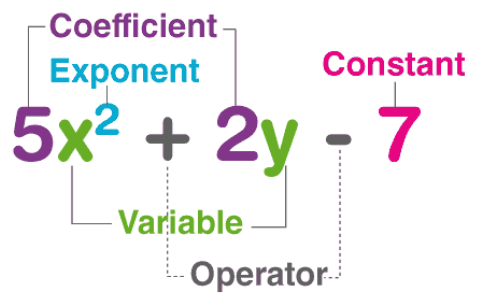Definition of a Polynomial

Let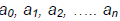be real numbers and x is a real variable. Then f(x) =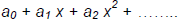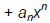is called a real polynomial of real variable x with coefficientsExample: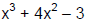is a polynomial

### Degree of a Polynomial

The degree of a polynomial is the highest power of the variable in the polynomial.

• Example: Degree of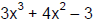is 3 as the maximum power of the variable x is 3.
• On the basis of degree, the polynomials are classified as Linear (Degree 1), Quadratic (degree 2), Cubical (Degree 3), bi-Quadratic (degree 4), and so on.

Question for Quadratic Equations: Introduction & Solved Examples
Try yourself:What is the degree of a polynomial of 4x7+9x5+5x2+11?

### Examples on Degree of a Polynomial

Q.1. Find the degree of the polynomial 6x4+ 3x2+ 5x +19

Ans: The degree of the polynomial is 4.

Q.2. Find the degree of the polynomial 6x3+4x3+3x+1

Ans: The degree of the polynomial is 3.

## Remainder Theorem

If any polynomial f(x) is divided by (x - a) then f(a) is the remainder.

• Example: f(x) = x2 - 5x + 7 = 0 is divided by x - 2. What is the remainder?
R = f(2) = 22 - 5 × 2 + 7 = 1.

## Factor Theorem

If (x - a) is a factor of f(x), then remainder f(a) = 0. (Or) if f(a) = 0, then (x - a) is a factor of f(x),

• Example: When f(x) = x2 - 5x + 6 = 0 is divided by x - 2, the remainder f(2) is zero which shows that x - 2 is the factor of f(x)

### Examples on Remainder & Factor Theorem

Q.1. Find the remainder by using the remainder theorem when polynomial x3−3x2+x+1 is divided by x−1.

Ans: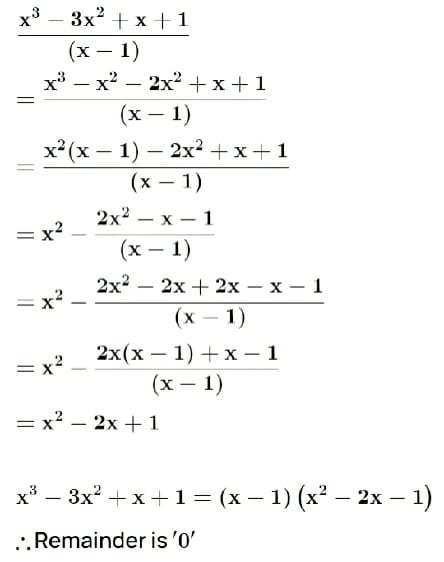Q.2. Find the remainder when p(x)= −x+3x2−1 is divided by x+1.

Ans: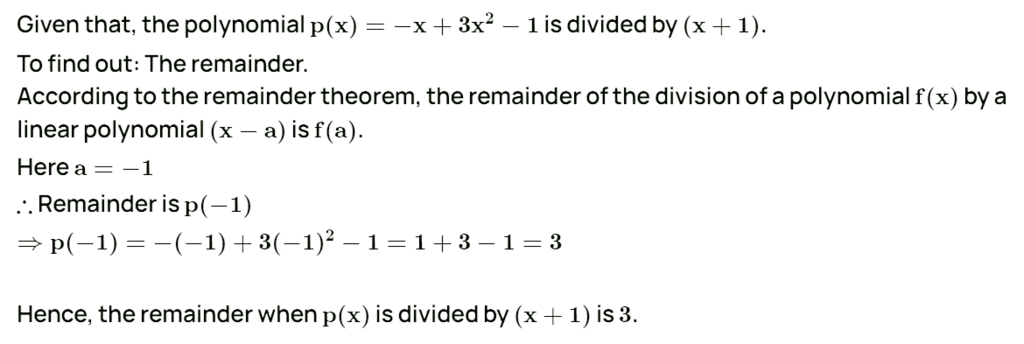## General Theory of Equations

• An equation is the form of a polynomial which has been equated to some real value.
• Example: 2x + 5 = 0, x2 - 2x + 5 = 7, 2x2 - 5x2 + 1 = 2x + 5 etc. are polynomial equations.

### Root or Zero of a Polynomial Equation

• If f (x) = 0 is a polynomial equation and f (α) = 0, then α is called a root or zero of the polynomial equation f(x) = 0.

## Linear Equation

### Linear Equation with One Variable

A linear equation is 1st degree equation. It has only one root. Its general form is a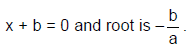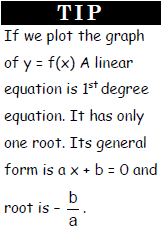### Linear Equation with Two Variables

• Example: 2x + 3y = 0. We need two equations to find the values of x and y.
If there are n variables in an equation, we need n equations to find the values of the variables uniquely.
• Sometimes, even the number of equations are equal to the number of variables, we cannot find the values of x and y uniquely.
For example, 3x + 5y = 6 & 6x +10y = 12
• These are two equations, but both are one and the same. So different values of x and y satisfy the equation and there is no unique solution. It will has infinite number of solutions.
• The number of solutions is clearly described below for the set of equations with 2 variables.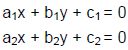• These equations can be:
(i) Inconsistent means have no solution if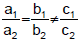(ii) Consistent and has infinitely many solutions if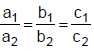(iii) Consistent and have unique solution if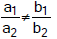Quadratic Equation in “x” is one in which the highest power of “x” is 2. The equation is generally satisfied by two values of “x”.

• The quadratic form is generally represented by ax2 + bx + c = 0 where a ≠ 0, and a, b, c are constants.
• For Example:
x2 - 6x + 4 = 0
3x2 + 7x - 2 = 0
• A quadratic equation in one variable has two and only two roots, which are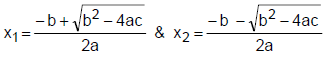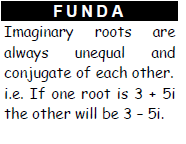### Nature of Roots

• The two roots of any quadratic equation always depend on the value of b2 - 4ac called discriminant (D).
(i) D > 0 Real and unequal roots
(ii) D = 0 Real and equal
(iii) D < 0 Imaginary and unequal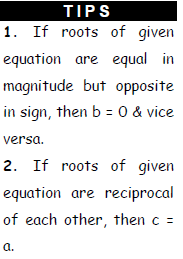Question for Quadratic Equations: Introduction & Solved Examples
Try yourself:Roots of a quadratic equation are imaginary when discriminant is:

### Sum and Product of Roots

• If α and β are the two roots of ax2 + bx + c = 0,
• Then sum of roots = α + β =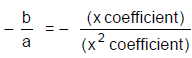• And product of roots = αβ =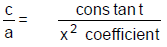### Formation of Equation from Roots

• If α and β are the roots of any quadratic equation then that equation can be written in the form: X2 − (α + β)X + αβ = 0
i.e. X2 - (sum of the roots) X + Product of the roots = 0

###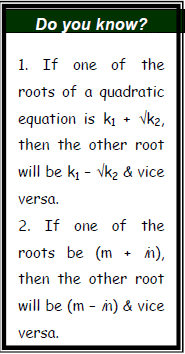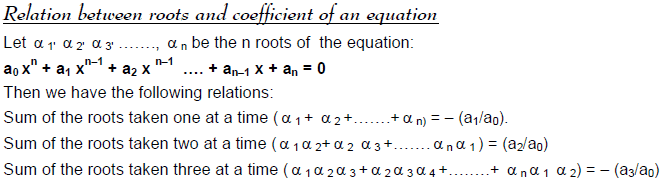-----------------------------------------------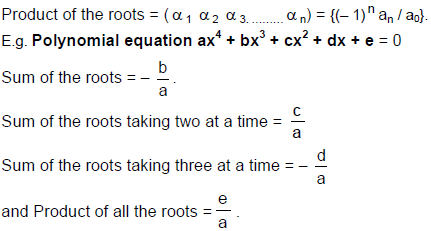Maximum and Minimum value of a Quadratic Equation

The quadratic equation ax2 + bx + c = 0 will have maximum or minimum value at x = - b/2a. If a < 0, it has maximum value and if a > 0, it has minimum value.

The maximum or minimum value is given by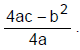### Solved Examples on Quadratic Equation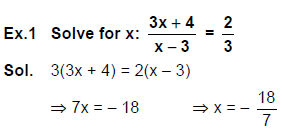Ex.2. A and B went to a hotel paid Rs. 84 for 3 plates of Idli and 5 plates of Dosa. Whereas B took 5 plates of Idli and 3 plates of Dosa and paid Rs. 76. What is the cost of one plate of Idli.

• 3I + 5D = 84 ……….(1)
• 5I + 3D = 76 ………(2)
• Equation (1) × 3 - equation (2) × 5, we get
⇒ 16I = 128
⇒ I = 8
• Each plate of Idli cost Rs. 8.

Ex.3. Find the values of x and y from the equations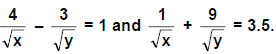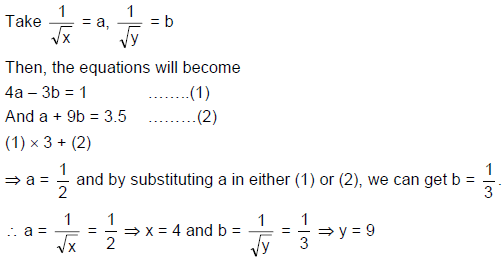Ex.4. Find x and y from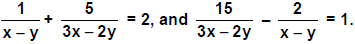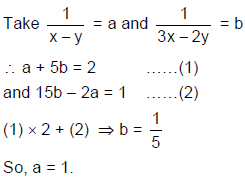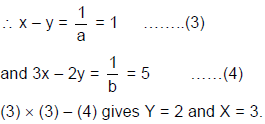Ex.5. Aman won a competition and so he got some prize money. He gave Rs. 2000 less than the half of prize money to his son and Rs. 1000 more than the two third of the remaining to his daughter. If both they got same amount, what is the prize money Aman got?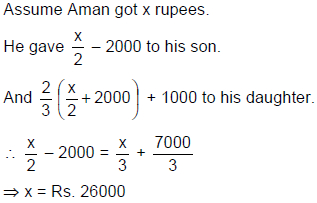Ex.6. How many non negative integer pairs (x, y) satisfy the equation, 3x + 4y = 21?

• Since x and y are non negative integers. Start from x = 0.
• If x = 0 or 2, y cannot be integer.
• For x = 3, y = 3.
And for x = 7, y = 0.
• These two pairs only satisfy the given equation.

Ex.7. If (x - 2) is a factor of x3 - 3x2 + px + 4. Find the value of p.

• Since (x - 2) is a factor, f(2) = 0.
• ∴ 23 - 3(22) + (2)p + 4 = 0
⇒ p = 0

Ex.8. When x3 - 7x2 + 3x - P is divided by x + 3, the remainder is 4, then what is the value of P ?

• f(- 3) = 4
• ∴ (- 3)3 - 7(- 3)2 + 3(- 3) - P = 4
• P = - 103.

Ex.9. If (x - 1) is the HCF of (x3 - px2 + qx - 3) and (x3 - 2x2 + px + 2). What is the value of ‘q’?

• Since (x - 1) is HCF, it is a factor for both the polynomials.
• ∴ 13 - p(1)2 + q(1) - 3 = 0
• - p + q = 2
• And 13 - 2(12) + p(1) + 2 = 0
• p = - 1
• ∴ q = 1

Ex.10. Find the roots of the quadratic equation x2 - x - 12 = 0.

• x2 - x - 12 = 0
• x2 - 4x + 3x - 12 = 0
• x(x - 4) + 3(x - 4) = 0
• (x - 4) (x + 3) = 0
• x = 4 or -3
• The roots are 4 and -3.
The document Quadratic Equations: Introduction & Solved Examples | General Test Preparation for CUET is a part of the CUET Course General Test Preparation for CUET.
All you need of CUET at this link: CUET

## General Test Preparation for CUET

177 videos|326 docs|399 tests

## FAQs on Quadratic Equations: Introduction & Solved Examples - General Test Preparation for CUET

 1. What is the definition of a polynomial?Ans. A polynomial is an algebraic expression consisting of variables, coefficients, and exponents, combined using addition, subtraction, and multiplication operations. It is a sum of terms, where each term is a product of a coefficient and one or more variables raised to non-negative integer exponents.
 2. What is the Remainder Theorem?Ans. The Remainder Theorem states that if a polynomial f(x) is divided by (x - a), the remainder is equal to f(a). In other words, if we substitute the value of 'a' into the polynomial, the resulting value will be the remainder when the polynomial is divided by (x - a).
 3. What is the Factor Theorem?Ans. The Factor Theorem states that if a polynomial f(x) has (x - a) as a factor, then f(a) = 0. In other words, if we substitute the value of 'a' into the polynomial and the resulting value is zero, then (x - a) is a factor of the polynomial.
 4. What is the General Theory of Equations?Ans. The General Theory of Equations is a branch of algebra that deals with the study of polynomial equations of any degree. It provides methods and techniques to solve these equations and find their roots. It encompasses various concepts like synthetic division, factorization, and the fundamental theorem of algebra.
 5. What are linear equations and how are they different from quadratic equations?Ans. Linear equations are polynomial equations of degree 1, meaning they involve variables raised to the power of 1. They can be expressed in the form ax + b = 0, where 'a' and 'b' are constants. Linear equations have a single solution. On the other hand, quadratic equations are polynomial equations of degree 2, meaning they involve variables raised to the power of 2. They can be expressed in the form ax^2 + bx + c = 0, where 'a', 'b', and 'c' are constants. Quadratic equations can have two solutions, known as roots, or no real solutions at all.

## General Test Preparation for CUET

177 videos|326 docs|399 testsExplore Courses for CUET examSignup to see your scores go up within 7 days! Learn & Practice with 1000+ FREE Notes, Videos & Tests.
10M+ students study on EduRev
Track your progress, build streaks, highlight & save important lessons and more!
Related Searches

,

,

,

,

,

,

,

,

,

,

,

,

,

,

,

,

,

,

,

,

,

;